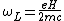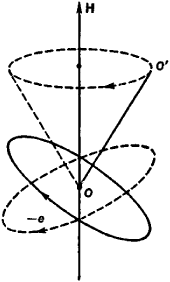# Larmor Precession

Also found in: Dictionary, Medical, Wikipedia.
Related to Larmor Precession: Larmor frequency

## Larmor precession

A precession in a magnetic field of the motion of charged particles or of particles possessing magnetic moments.

The Larmor theorem states that, for electrons moving in a single central field of force, the motion in a uniform magnetic field H is, to first order in H, the same as a possible motion in the absence of H except for the superposition of a common precession of angular frequency given by Eq. (1).

(1)Here e/c is the magnitude of the electronic charge in electromagnetic units, and m is the electronic mass. The frequency &ohgr;L is called the Larmor frequency and is numerically equal to 2&pgr; times 1.40 MHz per oersted or 2&pgr; times 111 MHz per SI unit of magnetic field strength (ampere-turn per meter). See Precession

In stating the Larmor theorem, use was made of the phrase “a possible motion.” If H is applied sufficiently slowly, it can be proved that the motion is the same as in the absence of H, except for the superposition of the Larmor precession. However, a sudden application of H may change, for example, a circular orbit into an elliptical one.

According to elementary electromagnetic theory, a current loop of area A and of current I possesses a magnetic moment μ of magnitude IA and of direction normal to the loop. Thus an electron moving in a circular orbit has an orbital magnetic moment.

The electron also has orbital angular momentum, which by quantum theory must equal &planck;J, where J is an integer and &planck; is Planck's constant h divided by 2&pgr;. In terms of the equivalent magnetic moment, Eq. (1) may be written in the form of

(2)Eq. (2). In this form the Larmor precession is exhibited by any magnetic moment μ including magnetic moments associated with spin angular momentum as well as those associated with orbital angular momentum. In this form the Larmor precession applies to experiments in molecular beams, electron paramagnetic resonance (EPR), and nuclear magnetic resonance (NMR). See Angular momentum, Electron spin, Magnetic resonance

## Larmor Precession

the supplementary rotation as a whole (”precession”) of a stable system of identical charged particles, such as the electrons in an atom, that occurs when a uniform, constant, relatively weak magnetic field is applied; the direction of the field is the axis of rotation. The existence of the precession was first demonstrated in 1895 by J. Larmor.

According to the Larmor theorem, when a uniform magnetic field H is applied, the equations of motion of a system of electrons are unchanged if one shifts to a system of coordinates that is rotating uniformly around the direction of the field with an angular velocity ωL = eH/2mc (in the absolute Gaussian system), where e and m are the charge and mass of an electron, respectively, and c is the velocity of light. The frequency ωL is known as the Larmor frequency. Thus, in the language of N. Bohr’s semiclassical theory of the atom, the magnetic field produces precession of the orbit of each atomic electron at a frequency ωL around the direction of the field (see Figure 1). Larmor precession is due to the action of the magnetic part of the Lorentz force on the charged particles and is analogous to the precession of the axis of a top (gyroscope) under the action of a force that tends to alter the direction of its axis of rotation.Figure 1. Precession of the orbit of an electron (with charge —e) in a magnetic field H; the axis of the orbit 00′ describes a cone around the direction of H

The Larmor theorem is valid if ωL is small compared to the natural frequencies of rotation of the particles in the absence of a magnetic field. For electrons even in very strong magnetic fields with H ~ 106 oersteds, ωL ~ 1013 sec−1, whereas the frequency of rotation of an electron in an atom is of the order (4Z2/n3)×1016sec−1 where Z is the charge on the nucleus and η is the principal quantum number; because of this the theorem has an extensive area of application. As a result of the supplementary rotation of the system of electrons in a magnetic field, a magnetic moment of the system appears. Consequently, the phenomenon of diamagnetism, the normal Zeeman effect, and the magnetic rotation of the plane of polarization may be explained on the basis of Larmor precession.

### REFERENCES

Becker, R. Elektronnaia teoriia. Moscow-Leningrad, 1936. (Translated from German.)
Landau, L. D., and E. M. Lifshits. Teoriia polia, 5th ed. Moscow, 1967. (Teoreticheskaia fizika, vol. 2.)

G. V. VOSKRESENSKII

## Larmor precession

[′lär·mȯr prē‚sesh·ən]
(electromagnetism)
A common rotation superposed upon the motion of a system of charged particles, all having the same ration of charge to mass, by a magnetic field.
References in periodicals archive ?
order because the Larmor precession that they induce is approximately
equivalent to Larmor precession in the classical case.
equivalent to the termination of the Larmor precession in the zero field
The fields are oriented in the correct sense in this case so that no [pi]-flipper is required between the two arms of the spectrometer as in a conventional (longitudinal field) NSE instrument; consequently Larmor precession occurs within the coil length in opposite directions on each side of the sample.
field component that is rotating counter to the direction of Larmor precession in the static field can be ignored.
In our method of the [pi] flipper phase scan with the three RF flippers setup, the resultant NSE pattern could be derived as one period of the [pi] flipper signal corresponding to two turns of the Larmor precession of neutrons in the apparatus .
3] He Self-Compensating Magnetometer," is to perform a high-precision test of combined charge conjugation, parity inversion, and time reversal (CPT) invariance and local Lorentz invariance by comparing the Larmor precession frequencies of potassium (K) and helium 3 ([He.

Site: Follow: Share:
Open / Close